# Count Number of Nodes

## Understanding

In this blog, we are going to analyze a problem based on Binary Trees. Binary Trees have a lot of interesting properties, and in this blog, we will have a greater understanding of these properties. Problems based on binary trees are prevalent in coding interviews and programming contests. This article will discuss various approaches to counting the number of nodes in a complete binary tree.

## The Problem Statement

Given the root of a complete binary tree, write a program to count the total number of nodes in the tree.

Now the question comes - What do you mean by a complete binary tree?

### Complete Binary Tree

A complete binary tree is a kind of binary tree that has all the levels filled except possibly the last one. In the last level as well, the nodes are as far left as possible. The number of nodes in the last level can be anything between 1 and 2^h, where h denotes the height of the last level.

The following examples will make the definition more clear.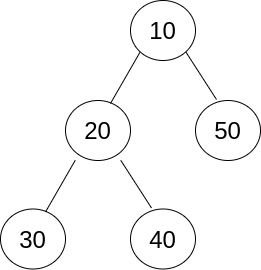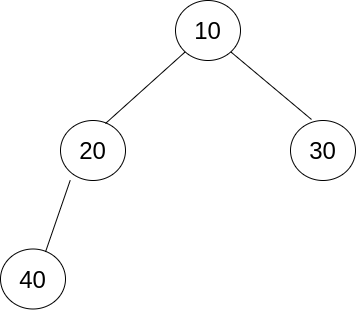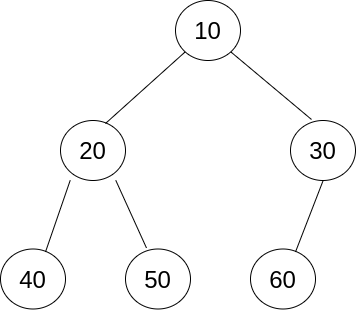Complete Binary Trees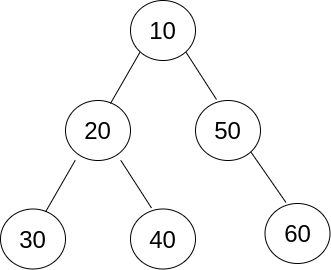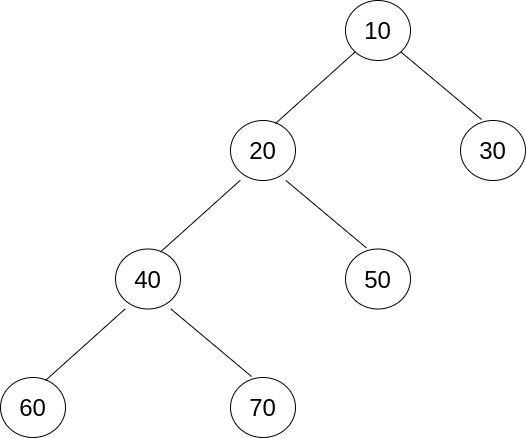Not Complete Binary Trees

### Input

Consider the complete binary tree in the image given as input to the program.### Output

Number of nodes: 6

Let us first look at the binary tree representation that we are going to follow in this article.

## Approach 1

A straightforward approach would be to traverse the whole binary tree and count the number of nodes as we encounter a new node. Recursion is the most common way to traverse a binary tree using pointers. The base case of the recursion procedure will evaluate if the tree node in consideration is null.

Let us discuss the algorithm now.

### Algorithm

1. Create the binary tree using the function defined in the struct Node structure.
2. Create a recursive function count_number_of_nodes, which takes a tree node as a parameter.
3. Base case: If the tree node is null, return 0.
4. Otherwise, return 1 + the sum of the number of nodes in the left subtree and right subtree.

### Output:

Number of nodes: 6

### Time Complexity

The time complexity of the above algorithm is O(n), where n is the number of nodes in the tree.

It is because the program traverses every node in the tree.

### Space Complexity

The space complexity of the above algorithm is O(log(n))

It is because the recursion can go at most log(n) deep. Hence the recursion stack can occupy O(log(n)) space in the worst case.

## Approach 2

Can we improve the time complexity? Surely yes. Notice that we have not yet used the definition of the complete binary tree.

Realize that we do not need to traverse the subtree of a tree node if we know that the leftmost node in the subtree is at the same depth as the rightmost node in the subtree. It would mean that the number of nodes in the subtree is equal to 2^h - 1, where h is the height of the tree node in consideration.

Let's code this approach.

### Algorithm

1. Create the binary tree using the function defined in the struct Node structure.
2. Left_height function: It takes a tree node as a parameter and calculates the depth of the leftmost node in the node's subtree. Putting it another way, this function returns the number of nodes in the path from the current node to the leftmost node in the current node's subtree.
3. Right_height function: It takes a tree node as a parameter and calculates the depth of the rightmost node in the node's subtree.
4. Create a recursive function that takes a tree node as a parameter. The base case of the function evaluates if the node is null.
5. Otherwise, if the depth of the leftmost and rightmost nodes in the current node's subtree are equal, return 2^h - 1 where h is the height of the current node.
6. If they are not equal, go by Approach 1.

### Output

Number of nodes: 6

### Time Complexity

The time complexity of the above algorithm is O(log(n) * log(n))

The time complexity of the left_height and right_height functions is log(n), where n is the number of nodes in the binary tree.

We calculate the height of the current node at each stage of the recursive procedure. And using the approach defined above, we need to consider only one node at each binary tree level. Why?

If the current node does not satisfy the proposed equality condition, then at least one of its children must satisfy the condition. It is because all the nodes in a complete binary are as far left as possible.

Since the height of a complete binary tree is of the order of O(log(n)), the total time complexity will be (log(n) * log(n)).

### Space Complexity

The space complexity of the above algorithm is the same as Approach 1, i.e., log(n).

In this approach as well, the depth of the recursion stack can be at most log(n) in the worst case.

## Key Takeaways

This article aimed at explaining different ways to count the number of nodes in a complete binary tree. I hope that the time complexity of Approach 2 is understandable. The algorithms used the concept of the height of a tree quite profoundly.

Yet learning never stops, and there is a lot more to learn.

So head over to our practice platform CodeStudio to practice top problems, attempt mock tests, read interview experiences, and much more. Till then, Happy Coding!

By Aman Chourasiya